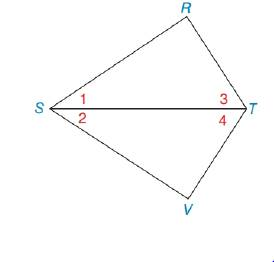Chapter 3.2, Problem 9E### Elementary Geometry for College St...

6th Edition
Daniel C. Alexander + 1 other
ISBN: 9781285195698

#### Solutions

Chapter
Section### Elementary Geometry for College St...

6th Edition
Daniel C. Alexander + 1 other
ISBN: 9781285195698
Textbook Problem
1 views

# In Exercise 5 to 12, plan and write the two-column proof for each problem. Given: ∠ R and ∠ V are right ∠ s . ∠ 1 ≅ ∠ 2Prove: Δ R S T ≅ Δ V S T

To determine

To prove:

The given statement.

Explanation

Given:

The following figure shows the given diagram.

Given that,

R and V are right s.

12

Approach:

By the definition of AAS, triangles will be congruent if two angles and non including side of one triangle are congruent to two angles and non including side of second triangle then both triangle must be congruent.

The completed proof is shown in the following table.

 Proof Statements Reasons 1. ∠R and ∠V are right ∠s and ∠1≅∠2 1. Given 2. ∠R≅∠V 2. All right angles are ≅ 3. ST¯≅ST¯ 3

### Still sussing out bartleby?

Check out a sample textbook solution.

See a sample solution

#### The Solution to Your Study Problems

Bartleby provides explanations to thousands of textbook problems written by our experts, many with advanced degrees!

Get Started

#### Find more solutions based on key concepts